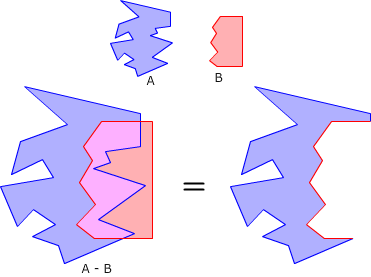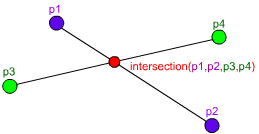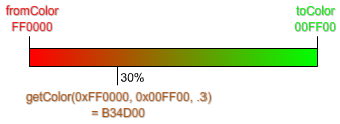## Archive for the ‘Math’ Category

### AS3 Polygon Clipper

Monday, May 23rd, 2011I was looking for an open source polygon clipper library for AS3 to use on a commercial project.
I found a few ports of the General Polygon Clipper library (GPC) but it is only free for non-commercial projects.
After more searching around I found this excellent library called Clipper by Angus Johnson. It did not have an AS3 port so I made one using Alchemy to wrap the c++ code.

The SWC and source code can be found on github: https://github.com/Flassari/as3clipper

It is completely free and open source for both personal and commercial projects. Clipper uses the Boost Software License.

Supported clipping operations: difference, intersection, union and XOR.

Here’s an example of how to use the AS3 port after importing the Clipper.swc file:

```import com.flassari.geom.Clipper;
import com.flassari.geom.ClipType;

var subjectPolygon:Array = [new Point(0, 0), new Point(200, 0), new Point(100, 200)];

var clipPolygon:Array = [new Point(0, 100), new Point(200, 100), new Point(300, 200)];

var resultPolygons:Array = Clipper.clipPolygon(subjectPolygon, clipPolygon, ClipType.DIFFERENCE);```

Update
There is now an AS3 port available at https://github.com/ChrisDenham/PolygonClipper.AS3

Friday, November 13th, 2009

Sometimes I have the need for a rotational progress bar that acts like a pie growing bigger (or smaller if that strikes your fancy). As usual, I made my own =)
The function drawPieMask takes first the graphics object of the displayObject instance and draws a part of pie on it, percentage set’s how big the part is.
If you want to offset the rotation of the pie (it starts to the right by default) you can set the rotation parameter. Note that rotation is in radians, not degrees, but you can multiply your degrees by (Math.PI/180) to convert to radians.
Lastly, the sides property determines how many sides the circle drawn in the mask has. You can see an example of different pie masks after the code.

To make the code as customizable as possible, it does not make a call to beginFill in case you want to set your own fill (or gradientfill even?).
If you just want to use it as a basic mask, just call beginFill(0) before and endFill() after the call to drawPieMask.

```function drawPieMask(graphics:Graphics, percentage:Number, radius:Number = 50, x:Number = 0, y:Number = 0, rotation:Number = 0, sides:int = 6):void {
// graphics should have its beginFill function already called by now
graphics.moveTo(x, y);
if (sides < 3) sides = 3; // 3 sides minimum
// Increase the length of the radius to cover the whole target
// Shortcut function
};
// Find how many sides we have to draw
var sidesToDraw:int = Math.floor(percentage * sides);
for (var i:int = 0; i <= sidesToDraw; i++)
lineToRadians((i / sides) * (Math.PI * 2) + rotation);
// Draw the last fractioned side
if (percentage * sides != sidesToDraw)
lineToRadians(percentage * (Math.PI * 2) + rotation);
}```

Example of different sides values. The last circle has a pie with 3 sides as a mask.

### Line-Line Intersection in AS3

Tuesday, April 28th, 2009I found a bug in my old Line-Line Intersection in C++ post, and after I fixed it I thought it would be a good idea to port it to AS3:

```function intersection(p1:Point, p2:Point, p3:Point, p4:Point):Point {
var x1:Number = p1.x, x2:Number = p2.x, x3:Number = p3.x, x4:Number = p4.x;
var y1:Number = p1.y, y2:Number = p2.y, y3:Number = p3.y, y4:Number = p4.y;
var z1:Number= (x1 -x2), z2:Number = (x3 - x4), z3:Number = (y1 - y2), z4:Number = (y3 - y4);
var d:Number = z1 * z4 - z3 * z2;

// If d is zero, there is no intersection
if (d == 0) return null;

// Get the x and y
var pre:Number = (x1*y2 - y1*x2), post:Number = (x3*y4 - y3*x4);
var x:Number = ( pre * z2 - z1 * post ) / d;
var y:Number = ( pre * z4 - z3 * post ) / d;

// Check if the x and y coordinates are within both lines
if ( x < Math.min(x1, x2) || x > Math.max(x1, x2) ||
x < Math.min(x3, x4) || x > Math.max(x3, x4) ) return null;
if ( y < Math.min(y1, y2) || y > Math.max(y1, y2) ||
y < Math.min(y3, y4) || y > Math.max(y3, y4) ) return null;

// Return the point of intersection
return new Point(x, y);
}```

You can try it here:

### Find the value between two colors

Wednesday, March 18th, 2009A handy function I wrote that calculates a color value between two colors.
```function getColor(fromColor:uint, toColor:uint, scale:Number):uint {
var red:uint = ((fromColor >> 16) + (toColor >> 16) - (fromColor >> 16) * scale) << 16;
var green:uint = ((fromColor >> 8 & 255) + ((toColor >> 8 & 255) - (fromColor >> 8 & 255)) * scale) << 8;
var blue:uint = (fromColor & 255) + ((toColor & 255) - (fromColor & 255)) * scale;

return red + green + blue;
}```

### Line-Line Intersection in C++

Monday, November 3rd, 2008I’ve just been tasked with creating a function to get the intersection of two lines.
With the help of equations from Wolfram Mathworld I created this nifty function:

```Point* intersection(Point p1, Point p2, Point p3, Point p4) {
// Store the values for fast access and easy
// equations-to-code conversion
float x1 = p1.x, x2 = p2.x, x3 = p3.x, x4 = p4.x;
float y1 = p1.y, y2 = p2.y, y3 = p3.y, y4 = p4.y;

float d = (x1 - x2) * (y3 - y4) - (y1 - y2) * (x3 - x4);
// If d is zero, there is no intersection
if (d == 0) return NULL;

// Get the x and y
float pre = (x1*y2 - y1*x2), post = (x3*y4 - y3*x4);
float x = ( pre * (x3 - x4) - (x1 - x2) * post ) / d;
float y = ( pre * (y3 - y4) - (y1 - y2) * post ) / d;

// Check if the x and y coordinates are within both lines
if ( x < min(x1, x2) || x > max(x1, x2) ||
x < min(x3, x4) || x > max(x3, x4) ) return NULL;
if ( y < min(y1, y2) || y > max(y1, y2) ||
y < min(y3, y4) || y > max(y3, y4) ) return NULL;

// Return the point of intersection
Point* ret = new Point();
ret->x = x;
ret->y = y;
return ret;
}```

Hope it will be to as good use to you as it is to me.